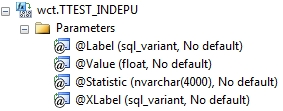# SQL Server independent samples T test

TTEST_INDEPU

Updated: 17 July 2012

Note: This documentation is for the SQL2008 (and later) version of this XLeratorDB function, it is not compatible with SQL Server 2005.

Use the aggregate function TTEST_INDEPU to perform a t-test on data in two samples, x and y assuming unequal variance. An independent samples t-test is used when you want to compare the means of a normally distributed interval dependent variable for two independent groups
The TTEST_INDEPU can return any of the following values.
P1           -              one-tailed probability
P2           -              two-tailed probability
T             -              t-statistic
DF           -              degrees of freedom
N1          -              number of observations (in the x group)
N2          -              number of observations (in the y group)
ES           -              effect size
MD         -              mean difference
SE           -              standard error
LCL         -              lower confidence level
UCL        -              upper confidence level
SyntaxArguments
@Label
the column variable which differentiates the two samples. You can use @Label to identify the control group and the treatment group.
@Value
the x-values and y-values to be used in the computation. @Value is an expression of type float or of a type that can be implicitly converted to float.
@Statistic
identifies the statistic to be returned.
@XLabel
Identifies which if the two values in the @Label column will be treated as x (or sample 1).
Return Types
float
Remarks
·         @Statistic must be invariant within a GROUP.
·         There must be exactly 2 values for @Label within a GROUP.
·         @XLabel must be invariant within a GROUP.
·         If @Value IS NULL it is not included in the aggregate.
·         TTEST_INDEPU is an aggregate function and follows the same conventions as all other aggregate function in SQL Server.
·         For paired t-test use TTEST_PAIRED.
·         For independent samples with equal variances use TTEST_INDEP.
·         Effect Size (ES) is calculated as Cohen’s d.
·         Unlike EXCEL and some other statistics packages, DF is not rounded before calculating the one-tailed or two-tailed p-values.
·         LCL and UCL are calculated with alpha = .05
Examples
Here’s a small sample of test scores broken out by gender.
SELECT wct.TTEST_INDEPU(x,val,'P1','F') as one_sided_p_value
FROM (VALUES
(1,'M',420),
(2,'M',647),
(3,'M',629),
(4,'M',569),
(5,'M',426),
(6,'F',554),
(7,'F',502),
(8,'F',580),
(9,'M',626),
(10,'F',412),
(11,'F',480),
(12,'M',478),
(13,'F',566),
(14,'F',491),
(15,'M',511),
(16,'F',556),
(17,'F',359),
(18,'F',451),
(19,'F',430),
(20,'M',441),
(21,'M',490),
(22,'M',578),
(23,'F',385),
(24,'M',592),
(25,'M',333)
) n(id, x, val)

This produces the following result.
one_sided_p_value
----------------------
0.140974751777123

If we wanted to calculate the t-statistic, we would just use T instead of P1
SELECT wct.TTEST_INDEPU(x,val,'T','F') as t_observed
FROM (VALUES
(1,'M',420),
(2,'M',647),
(3,'M',629),
(4,'M',569),
(5,'M',426),
(6,'F',554),
(7,'F',502),
(8,'F',580),
(9,'M',626),
(10,'F',412),
(11,'F',480),
(12,'M',478),
(13,'F',566),
(14,'F',491),
(15,'M',511),
(16,'F',556),
(17,'F',359),
(18,'F',451),
(19,'F',430),
(20,'M',441),
(21,'M',490),
(22,'M',578),
(23,'F',385),
(24,'M',592),
(25,'M',333)
) n(id, x, val)

This produces the following result.
t_observed
----------------------
-1.10263350525694

Here we show all the values that can be returned by TTEST_INDEPU.
SELECT t.s
,t.descr
,wct.TTEST_INDEPU(x,val,t.s,'F') as Value
FROM (VALUES
(1,'M',420),
(2,'M',647),
(3,'M',629),
(4,'M',569),
(5,'M',426),
(6,'F',554),
(7,'F',502),
(8,'F',580),
(9,'M',626),
(10,'F',412),
(11,'F',480),
(12,'M',478),
(13,'F',566),
(14,'F',491),
(15,'M',511),
(16,'F',556),
(17,'F',359),
(18,'F',451),
(19,'F',430),
(20,'M',441),
(21,'M',490),
(22,'M',578),
(23,'F',385),
(24,'M',592),
(25,'M',333)
) n(id, x, val)
CROSS APPLY(VALUES
('P1','one_tailed_p_value'),
('P2','two_tailed_p_value'),
('T','t_observed'),
('ES','effect_size'),
('N1','num_observed_one'),
('N2','num_observed_two'),
('DF','deg_freedom'),
('MD','mean_difference'),
('SE','std_error'),
('LCL','lower_ci'),
('UCL','upper_ci')
)t(s,descr)
GROUP BY t.s, t.descr
This produces the following result.
s    descr                               Value
---- ------------------ ----------------------
P1   one_tailed_p_value      0.140974751777123
P2   two_tailed_p_value      0.281949503554246
T    t_observed              -1.10263350525694
ES   effect_size            -0.436654132144636
N1   num_observed_one                       12
N2   num_observed_two                       13
DF   deg_freedom              22.2901656180061
MD   mean_difference         -37.9615384615385
SE   std_error                 34.428065427499
LCL  lower_ci                -109.307125545989
UCL  upper_ci                 33.3840486229124

### SupportCopyright 2008-2021 Westclintech LLC         Privacy Policy        Terms of Service